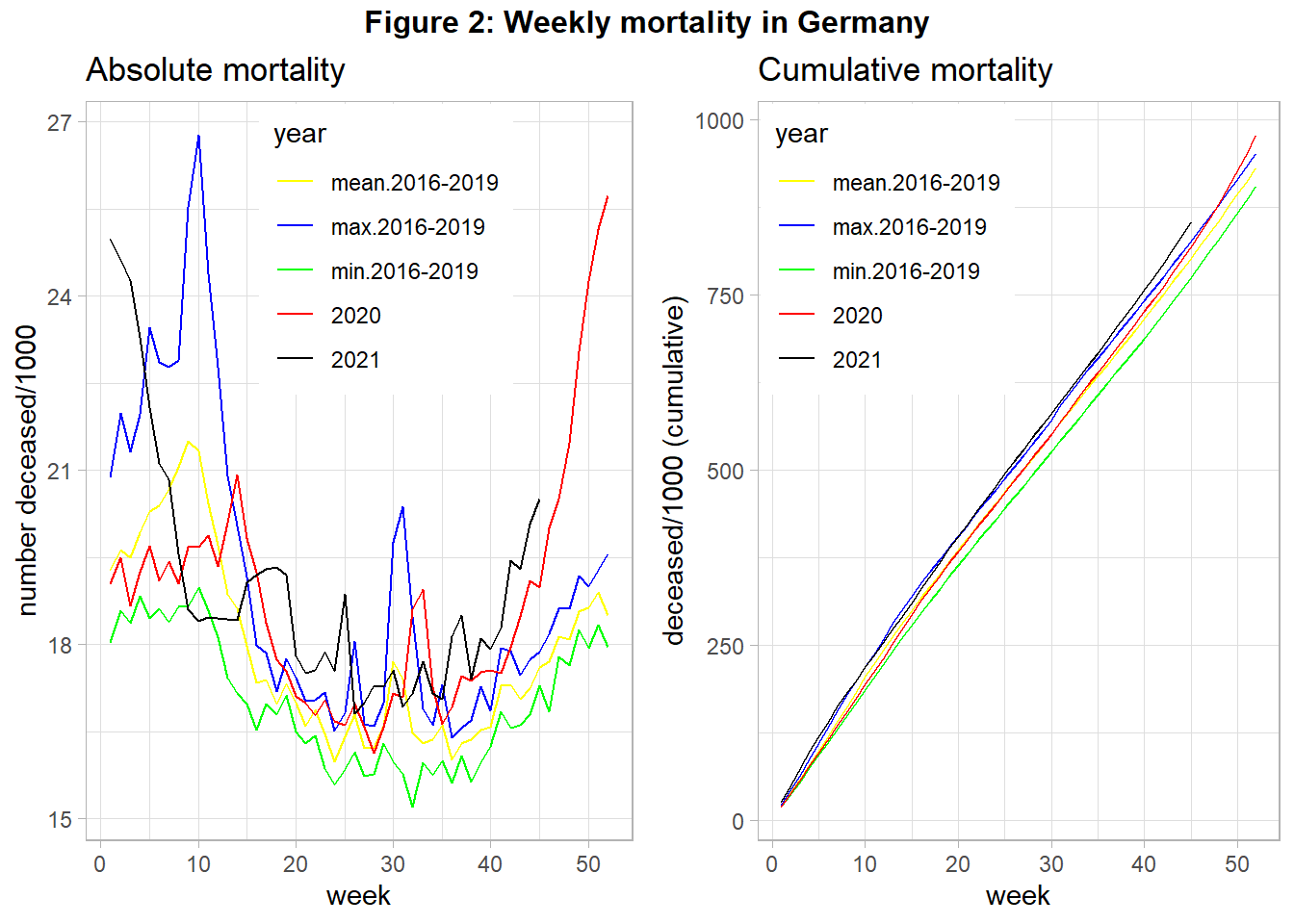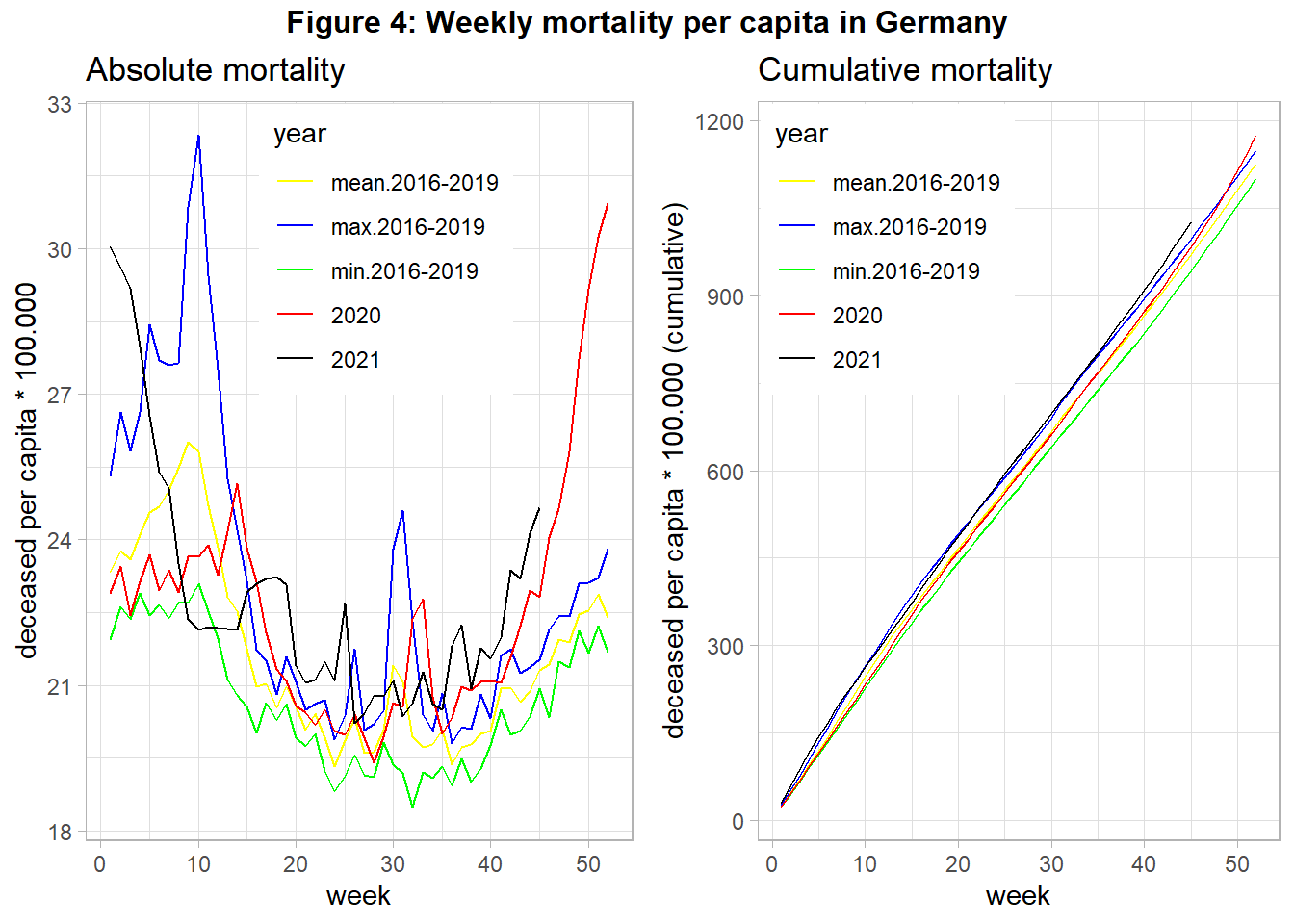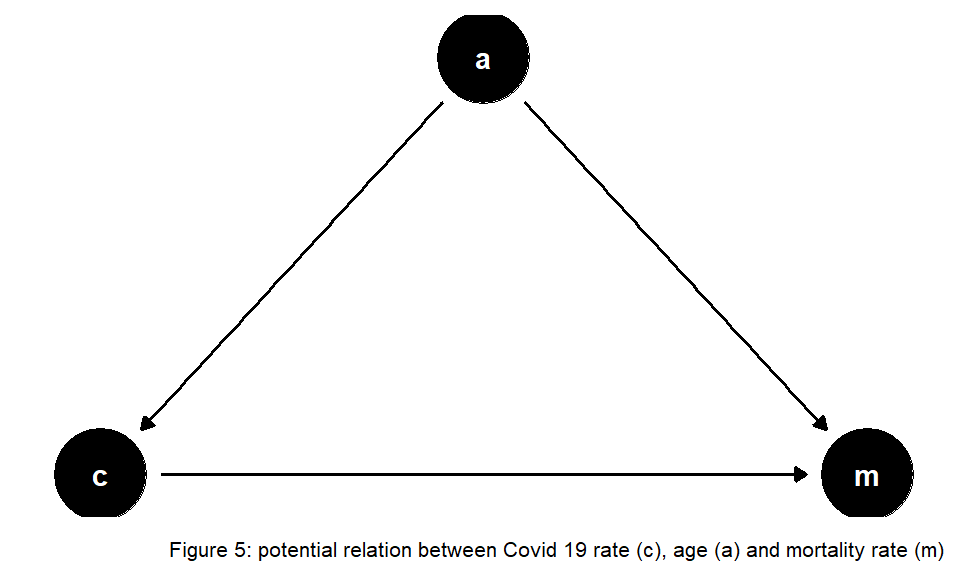Want to share your content on R-bloggers? click here if you have a blog, or here if you don't.

```#Preliminaries:

knitr::opts_chunk\$set( message=FALSE, warning=FALSE) #echo = FALSE,

rm(list=ls())

library(tidyverse)
library(gridExtra)
library(grid)
library(lubridate)

theme_set(theme_light())```

## Introduction

Since year 2019/2020 mankind has to deal with the communicable disease COVID-19. People across many countries and societies as a whole are affected from both an epidemiological and economic point of view. Data on mortality was regularly published across media, also to justify rigorous policy interventions. However, the numbers were rarely well explained. Hence, we close this gap by using public data from official statistics in order to visualize the mortality in Germany over time, also accounting for changes in population size. Following reproducible research paradigm, code is embedded within the document.

## Absolute Mortality

Figure 1 shows a simple relationship of total COVID-19 (C) and mortality (M) cases. If such a simple dependency holds then a comparison between then mortality in year 2020 with the preceding years may reveal the effect of Covid 19.Figure 2 shows the mortality of year 2020 (and 2021 up to November), in contrast to the mean-, minimum-, and maximum- mortality of the 4 preceding years.

```#read data
#source: https://www-genesis.destatis.de/

#source: https://www.destatis.de/DE/Themen/Gesellschaft-Umwelt/Bevoelkerung/Sterbefaelle-Lebenserwartung/Tabellen/sonderauswertung-sterbefaelle.html?nn=209016

pop_year <- pop %>%
#gather(`31.12.2015`, `31.12.2016`,`31.12.2017`,`31.12.2018`,`31.12.2019`,
#       key="end_of_year", value=population) %>%
mutate(year=as.integer(substr(Datum,5,8))+1) %>% #Population is taken as reference for following year
#group_by(Jahr) %>%
rename(population=Anzahl) %>%
select(year, population)

deaths_tab <- deaths %>%
#filter(Jahr<2021) %>%
gather(`01.01.`:`31.12.`,
key=day_month, value=deaths)  %>%
mutate(day=dmy(paste0(day_month, Jahr)), deaths=as.integer(str_replace(deaths, " ", ""))) %>%
rename(year=Jahr) %>%
select(year, day,deaths) %>%
drop_na(deaths)

#plot 1 absolute and 2 relative mortality (per capita)

#calculate number of deaths across time:
pop_death_time <-  deaths_tab %>%
group_by(year, week=week(day)) %>%
summarise(deaths=sum(deaths), days_in_week=n_distinct(day)) %>%
filter(days_in_week==7) %>%
group_by(year) %>%
arrange(week) %>%
mutate(deaths_cum=cumsum(deaths))  %>%
arrange(year, week) %>%
inner_join(pop_year, by="year") %>% ##add population data
mutate(deaths_rel=deaths/population, deaths_cum_rel=deaths_cum/population)

#subset data with year>=2020:
pop_death_time_2020 <- pop_death_time %>%
filter(year>=2020) %>%
select(year, week, deaths, deaths_cum, deaths_rel, deaths_cum_rel) %>%
mutate(year=as.character(year))

#subset time before 2020: min, mean, max death values (absolute and cumulative), same format as in pop_death_time_2020
pop_death_time_base <- pop_death_time %>%
filter(year<2020) %>%
group_by(week) %>%
summarise(mean_deaths=mean(deaths), min_deaths=min(deaths), max_deaths=max(deaths), mean_deaths_cum=mean(deaths_cum), min_deaths_cum=min(deaths_cum), max_deaths_cum=max(deaths_cum), mean_deaths_rel=mean(deaths_rel), min_deaths_rel=min(deaths_rel), max_deaths_rel=max(deaths_rel),
mean_deaths_cum_rel=mean(deaths_cum_rel), min_deaths_cum_rel=min(deaths_cum_rel), max_deaths_cum_rel=max(deaths_cum_rel)) %>%
gather(`min_deaths`, `max_deaths`, `mean_deaths`, `min_deaths_cum`, `max_deaths_cum`, `mean_deaths_cum`,
`min_deaths_rel`, `max_deaths_rel`, `mean_deaths_rel`, `min_deaths_cum_rel`, `max_deaths_cum_rel`, `mean_deaths_cum_rel`,
key="compare", value="death")  %>%
separate(compare, sep="_deaths", into=c("year", "outcome")) %>%
mutate(outcome=ifelse(outcome=="", "abs", outcome)) %>%
rename(deaths=abs, deaths_cum=`_cum`, deaths_rel=`_rel`, deaths_cum_rel=`_cum_rel`) %>%
relocate(year)

#data set containing information on >=2020,min,max,mean-mortality, both absolute and relative to population size
pop_death_time_comp <- pop_death_time_2020 %>%
bind_rows(pop_death_time_base) %>%
mutate(year=as_factor(case_when(year %in% c('2020', "2021") ~ year,
year=='max' ~ "max.2016-2019",
year=='min' ~ "min.2016-2019",
year=='mean' ~ "mean.2016-2019")), year=fct_relevel(year, "mean.2016-2019", "max.2016-2019", "min.2016-2019"))

#plot total deaths over time:
col <- c( "2020"="red", "2021"="black", "max.2016-2019"="blue", "mean.2016-2019"="yellow", "min.2016-2019"="green")

#1 plot absolute mortality
p1 <- pop_death_time_comp %>%
ggplot(aes(x=week, y=deaths/1000, group=year, color=year)) +
geom_line() +
scale_color_manual(values=col) +
labs(title = "Absolute mortality", y="number deceased/1000") +
theme(legend.position = c(0.55, 0.8))

#plot cumulative absolute mortality:
p2 <- pop_death_time_comp %>%
ggplot(aes(x=week, y=deaths_cum/1000, group=year, color=year)) +
geom_line() +
scale_color_manual(values=col) +
labs(title = "Cumulative mortality", y="deceased/1000 (cumulative)") +
theme(legend.position = c(0.25, 0.8))

#combine 2 plots next to each other
title1=textGrob("Figure 2: Weekly mortality in Germany", gp=gpar(fontface="bold"))
grid.arrange(p1,  p2, ncol=2, top=title1)          ```When interpreting this figure we have to acknowledge that in 2018 there was a very high mortality due to a very severe influenza season in the first quarter. We see for 2020 three peaks of relatively high mortality in week 15, around week 32, and from week 42 onward. The first and the last peak was correlated with strong policy interventions (“lockdown”). We also see that the cumulative mortality in 2020 was relatively high, at the end of the year even maximum mortality of year 2018 was surpassed. For year 2021 the mortality remains high, with an increasing tendency from the middle of the year onward.

## Mortality per capita

However these total number can provide wrong evidence about the mortality, if the population changes between the years: If the population number (P) increases we would expect that more people die (see Figure 3), also in absence of COVID-19.Hence, we have to account for change in population size. Figure 4 shows the data from above but uses the population of the beginning of each year in order to calculate number of deceased per capita.

```#2 Calculate Mortality per capita (population at the beginning of year):
#plot absolute mortality per capita:
p3 <- pop_death_time_comp %>%
ggplot(aes(x=week, y=deaths_rel*100000, group=year, color=year)) +
geom_line() +
scale_color_manual(values=col) +
labs(title = "Absolute mortality", y="deceased per capita * 100.000") +
theme(legend.position = c(0.55, 0.8))

#plot cumulative mortality per capita:
p4 <- pop_death_time_comp %>%
ggplot(aes(x=week, y=deaths_cum_rel*100000, group=year, color=year)) +
geom_line() +
scale_color_manual(values=col) +
labs(title = "Cumulative mortality", y="deceased per capita * 100.000 (cumulative)") +
theme(legend.position = c(0.25, 0.8))

#combine 2 plots next to each other
title2=textGrob("Figure 4: Weekly mortality per capita in Germany" , gp=gpar(fontface="bold"))
grid.arrange(p3,  p4, ncol=2, top=title2)```We find that changes of the number of citizens did not alter the found pattern from above. In the end of 2020 overall mortality increased strongly, probably driven by COVID-19. The same holds true for year 2021, although we have to keep in mind that this data is still preliminary. However, other potential relevant variables are not included into to the analysis. If the relationship between COVID-19 cases and mortality is confounded by the important variable age (see Figure 5) we need to account for changes in the age structure over the years in our analysis, as suggested in Figure 5:Empirical evidence suggests, after accounting for changes in age structure there is, overall, no increased mortality for the year 2020 in Germany (Ragnitz (2021)). However, the author argued that this was due to an decreased mortality during 2020 summer. These findings are supported by Theory meets practice… where also an analysis of age-specific mortality for Germany can be found. In contrast, the Federal Office of Statistics argues that increased mortality in Germany can be traced back to COVID-19, even after adjusting for changes in age composition. Also, it would be interesting to see the impact of COVID-19 on mortality if other important variables are taken into account.

So far, international comparisons suggest that some countries were more severely hit by the COVID-19 pandemic than others. The future may tell where this was due to better policies, and which other factors played an important role.

Take care and stay healthy! 🙂

## References

Ragnitz, Joachim. 2021. “Corona-Pandemie, Übersterblichkeit Und Der Lockdown Der Wirtschaft.” Ifo Dresden Berichtet 28 (01): 14–21.